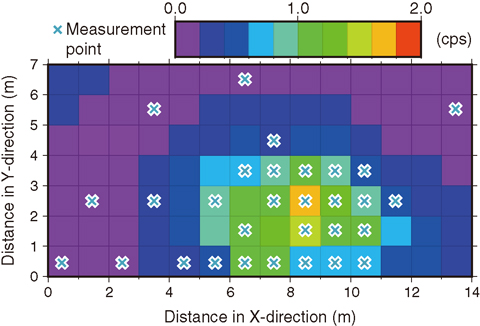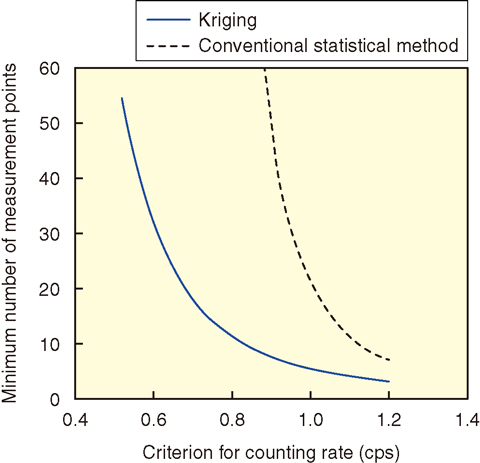# 2-6 Evaluation of Radioactivity Concentration for Site Release

## －Application of Kriging to the Evaluation of Mean Radioactivity Concentration with Consideration of Spatial Correlation－Fig.2-15　Example of the distribution of the radiation counting rate estimated by kriging

This is the distribution of the radiation counting rate including 30 measured data points (which were obtained in the region of relatively high count rates), as well as 68 data points that were estimated by kriging with consideration of spatial correlation based on the measured data. The mean counting rate estimated by kriging using all the data in the area is 0.41 cps, which is lower than that estimated by a conventional statistical method using only measured data, 0.71 cps.Fig.2-16　Minimum number of measurement points for the case of Fig.2-15, where the criterion for the counting rate is varied as a parameter

The minimum number of measurement points obtained by kriging is less than that obtained by a conventional statistical method. Since the difference between the estimated mean counting rate and the criterion value decreases with decreasing the criterion value, it is necessary to decrease the uncertainty in the estimated mean counting rate by increasing the minimum number of measurement points to ensure that the estimated mean counting rate including uncertainty is less than the criterion value.

After nuclear facilities are dismantled and demolished, the site (land and building) is deregulated for public use. This is called site release. A legal system regarding site release will be one of the important issues in the near future as the number of nuclear facilities such as nuclear power plants needing to be decommissioned increases. While the criterion and procedure regarding site release have not yet been established in Japan, it will eventually be necessary to confirm that significant radioactivity does not remain on the site before deciding to release it. In the United States, the standard manual of measurement and evaluation for site release has been published. It describes methods for ensuring compliance with the criterion for site release by evaluating the mean radioactivity concentration in the area of interest based on a limited amount of measured radioactivity data. In the evaluation, a conventional statistical method that does not consider spatial correlation of radioactivity concentrations between different points is applied. However, because radioactivity concentrations at the points generally have spatial correlation, it is recommended that the mean radioactivity concentration be evaluated with consideration of spatial correlation. Kriging, a geostatistics method, can estimate the value at a non-measuring point using measured data with consideration of spatial correlation.

Therefore, to support the measurement and evaluation, we studied a method of applying kriging to the estimation of the distribution of radioactivity concentration in the area of interest including non-measuring points, and the estimation of a mean concentration in the area from a limited amount of measured data. Fig.2-15 shows an example of a distribution of radiation counting rates estimated by kriging.

On the other hand, the estimated mean radioactivity concentration includes uncertainty, which may cause decision error by which the site may be judged to comply with the criterion of site release when the mean concentration actually exceeds the criterion value. The uncertainty decreases as the measurement points increase. To enhance the safety for site release, it is necessary to calculate the uncertainty and to determine a minimum number of measurement points corresponding to a given low probability of decision error. Therefore, we proposed a method to calculate the uncertainty in the estimated mean radioactivity concentration as a function of the number of measurement points and to calculate the minimum number of measurement points corresponding to a given probability of decision error.

Fig.2-16 shows the minimum number of measurement points for the case of Fig.2-15, where the criterion for the counting rate is varied as a parameter. It shows that the minimum number of measurement points obtained by kriging was less than that by a conventional statistical method.

The outcome obtained in this study will contribute to the establishment of a criterion regarding site release for nuclear facilities in the near future.

| | | | |